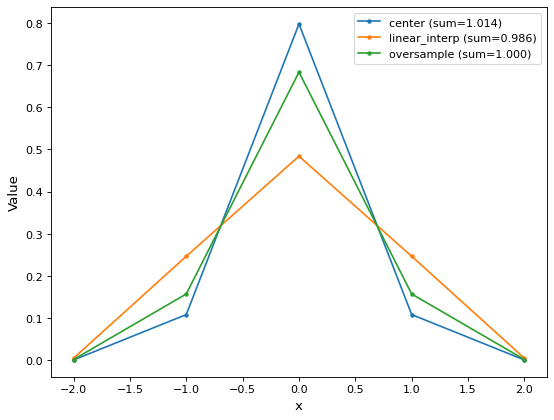# discretize_model¶

`astropy.convolution.``discretize_model`(model, x_range, y_range=None, mode='center', factor=10)[source]

Function to evaluate analytical model functions on a grid.

So far the function can only deal with pixel coordinates.

Parameters
model`Model` or callable.

Analytic model function to be discretized. Callables, which are not an instances of `Model` are passed to `custom_model` and then evaluated.

x_range`tuple`

x range in which the model is evaluated. The difference between the upper an lower limit must be a whole number, so that the output array size is well defined.

y_range`tuple`, optional

y range in which the model is evaluated. The difference between the upper an lower limit must be a whole number, so that the output array size is well defined. Necessary only for 2D models.

mode`str`, optional
One of the following modes:
• `'center'` (default)

Discretize model by taking the value at the center of the bin.

• `'linear_interp'`

Discretize model by linearly interpolating between the values at the corners of the bin. For 2D models interpolation is bilinear.

• `'oversample'`

Discretize model by taking the average on an oversampled grid.

• `'integrate'`

Discretize model by integrating the model over the bin using `scipy.integrate.quad`. Very slow.

factor

Factor of oversampling. Default = 10.

Returns
array`numpy.array`

Model value array

Notes

The `oversample` mode allows to conserve the integral on a subpixel scale. Here is the example of a normalized Gaussian1D:

```import matplotlib.pyplot as plt
import numpy as np
from astropy.modeling.models import Gaussian1D
from astropy.convolution.utils import discretize_model
gauss_1D = Gaussian1D(1 / (0.5 * np.sqrt(2 * np.pi)), 0, 0.5)
y_center = discretize_model(gauss_1D, (-2, 3), mode='center')
y_corner = discretize_model(gauss_1D, (-2, 3), mode='linear_interp')
y_oversample = discretize_model(gauss_1D, (-2, 3), mode='oversample')
plt.plot(y_center, label='center sum = {0:3f}'.format(y_center.sum()))
plt.plot(y_corner, label='linear_interp sum = {0:3f}'.format(y_corner.sum()))
plt.plot(y_oversample, label='oversample sum = {0:3f}'.format(y_oversample.sum()))
plt.xlabel('pixels')
plt.ylabel('value')
plt.legend()
plt.show()
```

(png, svg, pdf)